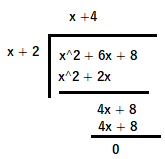mycollegehive
A rectangular prism has a volume of 3x^2 + 18x + 24. Its base has a length of x + 2 and a width of 3. Which expression represents the height of the prism?
JMAP math geometry0A rectangular prism has a volume of 3x2 + 18x + 24. Its base has a length of x + 2 and a width of 3. Which expression represents the height of the prism?

1) x + 4

2) x + 2

3) 3

4) x2 + 6x + 8

58 viewsShareFollowUniversity of Benin Nigeria
11 June 2020University of Lagos Nigeria
12 August 20200The volume of any prism can also be given as the base area of that prism multiplied by the height. That is,

Volume of prism = Area of base x Height of prism

for a rectangular base,

Area of base = Length x Width.

Thus, volume of the rectangular prism = Length x Width x Height

3x2 + 18x + 24 = (x + 2) * 3 * h

divide through by 3

x2 + 6x + 8 = (x + 2)h

Divid both sides by x+2

h = (x2 + 6x + 8) / (x + 2)from division of polynomial, h = x + 4

Ans 1Share### Related Tags

JMAP

2 followers

143 questionsmath

8 followers

1015 questionsgeometry

1 followers

99 questions